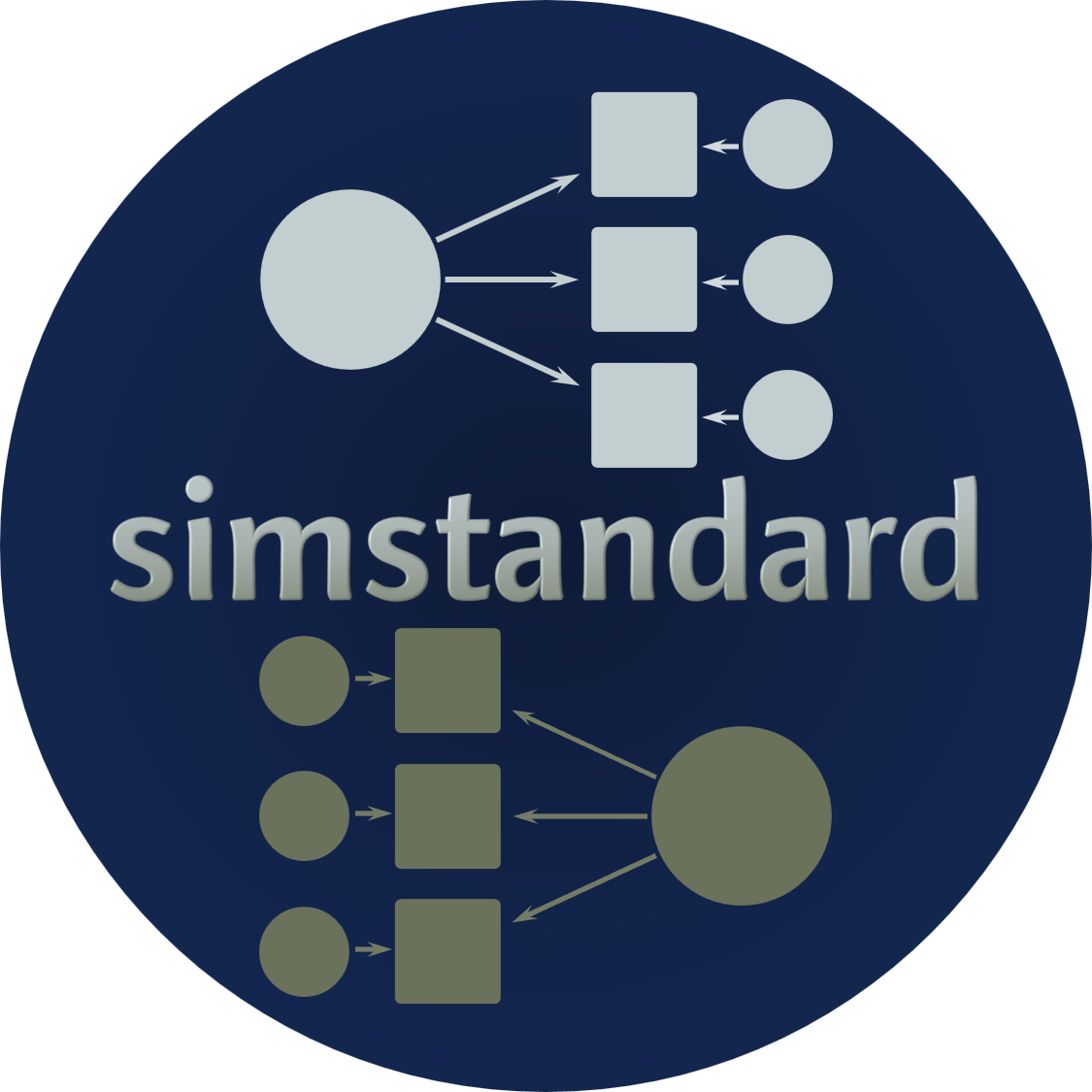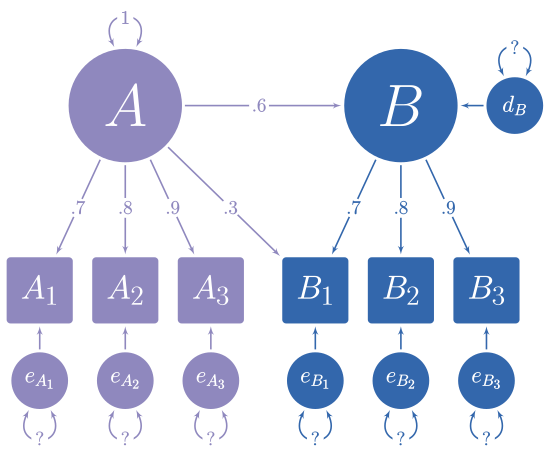# Using the simstandard package

#### 2020-10-22# Purpose of simstandard

In the figure below, you can see a hypothetical structural model with its standardized loadings and path coefficients.Suppose you need to simulate multivariate normal data based on this model, but you do not know the error variances and the latent disturbance variances needed to make your model produce standardized data. It is often difficult to find such values algebraically, and instead they must be found iteratively.

The simstandard package finds the standardized variances and creates standardized multivariate normal data using lavaan syntax. It can also create latent variable scores, error terms, disturbance terms, estimated factor scores, and equally weighted composite scores for each latent variable.

# Generate Model-Based Multivariate Data

``````library(simstandard)
library(lavaan)
library(knitr)
library(dplyr)
library(ggplot2)
library(tibble)
library(tidyr)

# lavaan syntax for model
m <- "
A =~ 0.7 * A1 + 0.8 * A2 + 0.9 * A3 + 0.3 * B1
B =~ 0.7 * B1 + 0.8 * B2 + 0.9 * B3
B ~ 0.6 * A
"

# Simulate data
d <- sim_standardized(m, n = 100000)

# Display First 6 rows
A1 A2 A3 B1 B2 B3 A B e_A1 e_A2 e_A3 e_B1 e_B2 e_B3 d_B
1.51 0.88 1.03 2.38 3.58 2.87 1.31 2.79 0.60 -0.17 -0.15 0.04 1.35 0.36 2.00
1.57 0.59 0.51 0.33 0.51 1.12 1.05 0.68 0.83 -0.26 -0.43 -0.46 -0.03 0.51 0.05
-0.50 0.59 0.76 0.36 -0.31 0.70 0.04 0.17 -0.52 0.56 0.73 0.23 -0.45 0.55 0.15
-0.27 -0.94 -0.76 -0.29 0.22 -0.68 -0.86 -0.46 0.33 -0.26 0.01 0.29 0.58 -0.27 0.06
-0.02 -0.81 0.45 -0.23 0.72 0.20 1.04 -0.27 -0.75 -1.65 -0.49 -0.35 0.94 0.44 -0.89
-1.44 -0.19 -0.79 -1.13 -0.44 -0.82 -0.97 -0.67 -0.77 0.58 0.07 -0.37 0.09 -0.22 -0.09

Let’s make a function to display correlations and covariance matrices:

``````ggcor <- function(d) {
require(ggplot2)
as.data.frame(d) %>%
tibble::rownames_to_column("rowname") %>%
tidyr::gather(colname, r, -rowname) %>%
dplyr::mutate(rowname = forcats::fct_rev(rowname)) %>%
dplyr::mutate(colname = factor(colname,
levels = rev(levels(rowname)))) %>%
ggplot(aes(colname, rowname, fill = r)) +
geom_tile(color = "gray90") +
geom_text(aes(label = formatC(r, digits = 2, format = "f") %>%
stringr::str_replace_all("0\\.",".") %>%
stringr::str_replace_all("1.00","1")),
color = "white",
fontface = "bold",
family = "serif") +
na.value = "gray20",
limits = c(-1.01, 1.01),
high = "#924552",
low = "#293999") +
coord_equal() +
scale_x_discrete(NULL,position = "top") +
scale_y_discrete(NULL) +
theme_light(base_family = "serif", base_size = 14)
}``````

Because the data are standardized, the covariance matrix of the observed and latent variables should be nearly identical to a correlation matrix. The error and disturbance terms are not standardized.

``````cov(d) %>%
ggcor``````To return only the observed variables

``````d <- sim_standardized(m,
n = 100000,
latent = FALSE,
errors = FALSE)
# Display First 6 rows
A1 A2 A3 B1 B2 B3
1.61 1.15 2.06 0.90 -0.05 -0.08
1.46 1.50 0.68 0.12 -0.48 0.34
-0.89 -1.19 -1.22 -0.52 0.40 -0.73
0.13 -1.55 -0.79 -1.00 -0.17 -1.18
-0.93 -1.64 -0.96 -1.23 -1.51 -1.01
-0.43 -0.24 -0.10 -0.87 -0.78 -1.21

# Comparison with `lavaan::simulateData`

I love the lavaan package. However, one aspect of one function in lavaan is not quite right yet. lavaan’s `simulateData` function is known to generate non-standardized data, even when the `standardized` parameter is set to `TRUE`. See how it creates variable Y with a variance higher than 1.

``````test_model <- "
Y ~ -.75 * X_1 + .25 * X_2
X =~ .75 * X_1 + .75 * X_2
"

library(lavaan)
d_lavaan <- simulateData(
model = test_model,
sample.nobs = 100000,
standardized = TRUE)
cov(d_lavaan) %>%
ggcor``````With the same test model, simstandard will calculate variables with variances of 1.

``````sim_standardized(test_model,
n = 100000,
errors = FALSE) %>%
cov %>%
ggcor()``````# Inspecting model matrices

You can inspect the matrices that simstandard uses to create the data by calling `simstandardized_matrices`.

``matrices <- sim_standardized_matrices(m)``

The A matrix contains all the asymmetric path coefficients (i.e., the loadings and the structural coefficients). These coefficients are specified in the lavaan model syntax.

``````matrices\$RAM_matrices\$A %>%
ggcor()``````The S matrix contains all the symmetric path coefficients (i.e., the variances and correlations of the observed and latent variables). For endogenous variables, the variances and correlations refer to the variance and correlations of the variable’s associated error or disturbance term. In this case, A is the only endogenous variable, and thus its variance on the diagonal of the S matrix is 1.

``````matrices\$RAM_matrices\$S %>%
ggcor()``````